# A note on the volumetric-deviatoric split on the anisotropic constitutive model for fiber-reinforced materials

https://doi.org/10.33263/BioMed12.016024

Tao Jin 1and Ilinca Stanciulescu 1,*

1  Rice University, Department of Civil and Environmental Engineering, Houston, United States; taojin83@gmail.com

* Correspondence: ilinca.s@rice.edu; Scopus ID: 12790619700

## Abstract

In the literature, it has been suggested that for a class of anisotropic constitutive laws for fiber-reinforced materials, the volumetric-deviatoric split should only be performed on the isotropic (matrix) term, but not on the anisotropic (fiber) term. In this research note, we follow up on the theoretical and numerical analyses adopted in these early publications with an intuitive example that allows us to directly analyze the effect of this split. We demonstrate that performing such split on the anisotropic term leads to non-physical volume growth of the material sample. Therefore, we consolidate the observation that the volumetric-deviatoric split should not be applied to the anisotropic (fiber) term of the total strain energy.

## 1. Introduction

Biological tissues, such as ligament, skin and arterial wall, are often categorized as fiber-reinforced materials and can be modeled with two components, the non-collagenous matrix and the collagen fibers [1-6]. The fibers are usually much stiffer than the surrounding matrix, making the material anisotropic with the preferred direction following the mean orientation of the distributed collagen fibers. Among different types of constitutive formulations for fiber-reinforced materials, a common choice is to adopt an isotropic (matrix) strain energy function enhanced with an anisotropic (fiber) term. In the anisotropic term, the material anisotropy is represented via a structural tensor .

It is common to decouple the isotropic strain energy of fiber-reinforced materials into the volumetric and deviatoric contributions. When Poisson’s ratio is close to 0.5, the volumetric term containing the bulk modulus $\mathrm{K}$ serves as a penalty to ensure that the material satisfies the nearly incompressibility [7, 8]. However, the volumetric-deviatoric split on the anisotropic strain energy is inconsistently used in the literature. For example, in some cases the full pseudo-invariant $\bar{\mathrm{I}_\mathrm{4}}$ is adopted in the anisotropic term [3, 9-11], while in other cases only its deviatoric part$\mathrm{I}_\mathrm{4}$ is used [1, 4, 12, 13]. Sansour  argued that the volumetric-deviatoric split should not be performed on the anisotropic (fiber) part, otherwise the assumption that the change of material volume only depends on a spherical state of stress would be violated. Helfenstein et al.  reached a similar conclusion by numerically demonstrating that nearly incompressible materials would undergo non-physical volume growth if the anisotropic term depends only on the deviatoric deformation. We follow up on the theoretical and numerical analyses adopted in these early publications with an intuitive example that allows us to directly analyze the effect of this split. Using both analytical solutions and computational results, we consolidate the observation that the volumetric-deviatoric split should not be applied to the anisotropic (fiber) term of the total strain energy.

## 2. Constitutive modeling

A common approach to model fiber-reinforced materials is to smear the fiber component in the surrounding matrix and treat the material as homogeneous. The total strain energy function is decoupled into two terms$\mathrm{\psi=}{\mathrm{\psi}}_{\mathrm{iso}}\mathrm{+}{\mathrm{\psi}}_{\mathrm{aniso}}$

(1)

where the isotropic term ${\mathrm{\psi}}_{\mathrm{iso}}$ and the anisotropic term $\mathrm{\psi}_{\mathrm{aniso}}$represent the contributions from the matrix and the fiber component. In$\mathrm{\psi}_{\mathrm{aniso}}$, the structural tensor$\mathrm{A=N}\mathrm{\bigotimes}\mathrm{N}$ is included to capture the material anisotropy, where $\mathrm{N}$ is a unit directional vector representing the fiber orientation. In order to guarantee the frame-indifference of the constitutive model,$\mathrm{\psi}_{\mathrm{aniso}}$ is written as a function containing a pseudo-invariant of the structural tensor $\mathrm{A}$, for example, the full invariant [3, 9-11]$\mathrm{I}_\mathrm{4}\mathrm{=C∶A,}$

(2)

or its deviatoric part [1, 4, 12, 13]

$\bar{\mathrm{I}_\mathrm{4}}\mathrm{=}\bar{\mathrm{C}}\mathrm{∶A=}\mathrm{J}^{\mathrm{-}\frac{\mathrm{2}}{\mathrm{3}}}\mathrm{C:A,}$

(3)

where $\mathrm{C=F}^\mathrm{T}\mathrm{F}$ is the right Cauchy-Green tensor, and $\mathrm{J=detF}$ is the determinant of the deformation gradient $\mathrm{F}$. The isotropic matrix is described by the modiffed neo-Hookean model,

${\mathrm{\ \psi}}_{\mathrm{m}}{=}\mathrm{\psi}_{\mathrm{vol}}\left(\mathrm{J}\right)\mathrm{+}{\mathrm{\ \psi}}_{\mathrm{dev}}\left(\mathrm{\bar{\mathrm{I}_\mathrm{1}}}\right)\mathrm={\frac{\mathrm{1}}{\mathrm{4}}}K\left(\mathrm{{J}^{\mathrm{2}}\mathrm{-1-2ln{J}}}\right)\mathrm{+}{\frac{\mathrm{1}}{\mathrm{2}}}G\left(\bar{\mathrm{I}_\mathrm{1}}\mathrm {-3}\right),$

(4)

where $K = \frac{\mathrm{E}}{\mathrm{3(1-2\nu)}}$is the bulk modulus, $G = \frac{\mathrm{E}}{\mathrm{2(1+\nu)}}$ is the shear modulus, $\mathrm{E}$ is Young’s modulus, ν is Poisson’s ratio, and$\bar{\mathrm{I}_\mathrm{1}}\mathrm{=J}^{\mathrm{-}\frac{\mathrm{2}}{\mathrm{3}}}\mathrm{tr}\left(\mathrm{F}^\mathrm{T}\mathrm{F}\right)$ is the modified tensor invariant. In order to directly compare the influence of using $\mathrm{I}_\mathrm{4}$ or $\bar{\mathrm{I}_\mathrm{4}}$ in the anisotropic strain energy$\mathrm{\psi}_{\mathrm{aniso}}$, the exponential form proposed by Holzapfel et al.  is adopted in this research.

### 2.1. Anisotropic term using $\mathrm{I}_\mathrm{4}$

When the full pseudo-invariant of the structural tensor $\mathrm{I}_\mathrm{4}$ is used, the anisotropic strain energy of the Holzapfel-Gasser-Ogden (HGO) model is written as

$\mathrm{\psi}_{\mathrm{aniso}}\mathrm{\ =\ }\mathrm{\psi}_{\mathrm{aniso}}^{\left(\mathrm{1}\right)}\left(\mathrm{I}_\mathrm{4}\right)\mathrm{=}{\frac{\mathrm{\mathrm{k}_{\mathrm{1}}}}{\mathrm{2\mathrm{k}_{\mathrm{2}}}}}\left(\mathrm{e}^{\mathrm{\mathrm{k}_\mathrm{2}}\left(\mathrm{I}_\mathrm{4}\mathrm{-1}\right)^{\mathrm{2}}}-1\right),$

(5)

where $\mathrm{k}_\mathrm{1}$ is a stress-like material parameter, and $\mathrm{k}_\mathrm{2}$ is a dimensionless parameter. The 2nd Piola-Kirchhoff stress is derived from Equation (5) as

$\mathrm{S}_{\mathrm{aniso}}^{\left(\mathrm{1}\right)}\mathrm{=2}\frac{\mathrm{\partial}\mathrm{\psi}_{\mathrm{aniso}}^{\left(\mathrm{1}\right)}\mathrm{(} \mathrm{I}_\mathrm{4}\mathrm{)} }{\mathrm{\partial}\mathrm{C}}\mathrm{=2}\mathrm{k}_\mathrm{1}\left(\mathrm{I}_\mathrm{4}\mathrm{-1} \right)\mathrm{e}^{\mathrm{k}_\mathrm{2}{\mathrm{(}\mathrm{I}_\mathrm{4}\mathrm{-1)} }^\mathrm{2}}\mathrm{A}.$

(6)

The corresponding Cauchy stress is

$\mathrm{\sigma}_{\mathrm{aniso}}^{\left(\mathrm{1}\right)}\mathrm{=}\mathrm{J}^{\mathrm{-1}}\mathrm{F}\mathrm{S}_{\mathrm{aniso}}^{\left(\mathrm{1}\right)}\mathrm{F}^{\mathrm{T}}{=}\mathrm{2}\mathrm{k}_\mathrm{1}\left(\mathrm{I}_\mathrm{4}\mathrm{-1}\right)\mathrm{e}^{\mathrm{k}_\mathrm{2}{\mathrm{(}\mathrm{I}_\mathrm{4}\mathrm{-1)} }^\mathrm{2}}\mathrm{J}^{\mathrm{-1}}\mathrm{n\bigotimes n},$

(7)

where $\mathrm{n}\mathrm{=}\mathrm{FN}$ is the fiber orientation in the deformed configuration. Equation (7) shows that using $\mathrm{I}_\mathrm{4}$ in the anisotropic strain energy $\mathrm{\psi}_{\mathrm{aniso}}$ leads to a stress state aligned with the deformed fiber orientation.

### 2.2. Anisotropic term using$\bar{\mathrm{I}_\mathrm{4}}$

When the deviatoric part of the pseudo invariant of the structural tensor $\bar{\mathrm{I}_\mathrm{4}}$is used, the anisotropic strain energy of the HGO model is written as

$\mathrm{\psi}_{\mathrm{aniso}}\mathrm{\ =\ }\mathrm{\psi}_{\mathrm{aniso}}^{\left(\mathrm{2}\right)}\left(\bar{\mathrm{I}_\mathrm{4}}\right)\mathrm{=}{\frac{\mathrm{\mathrm{k}_{\mathrm{1}}}}{\mathrm{2\mathrm{k}_{\mathrm{2}}}}}\left(\mathrm{e}^{\mathrm{\mathrm{k}_\mathrm{2}}\left(\bar{\mathrm{I}_\mathrm{4}}\mathrm{-1}\right)^{\mathrm{2}}}-1\right),$

(8)

The 2nd Piola-Kirchhoff stress in the reference configuration can be derived from Equation (8) as

$\mathrm{S}_{\mathrm{aniso}}^{\left(\mathrm{2}\right)}\mathrm{=2}\frac{\mathrm{\partial}\mathrm{\psi}_{\mathrm{aniso}}^{\left(\mathrm{2}\right)}\mathrm{(}\bar{\mathrm{I}_\mathrm{4}}\mathrm{)} }{\mathrm{\partial}\mathrm{C}}\mathrm{=2}\mathrm{k}_\mathrm{1}\left(\bar{\mathrm{I}_\mathrm{4}}\mathrm{-1} \right)\mathrm{e}^{\mathrm{k}_\mathrm{2}{\mathrm{(}\bar{\mathrm{I}_\mathrm{4}}\mathrm{-1)} }^\mathrm{2}}\left(\mathrm{J}^{\mathrm{-}\frac{\mathrm{2}}{\mathrm{3}}}\mathrm{A}\mathrm{-}\frac{\mathrm{1}}{\mathrm{3}}\bar{\mathrm{I}_\mathrm{4}}\mathrm{C}^{\mathrm{-1}}\right).$

(9)

The corresponding Cauchy stress in the deformed configuration is

$\mathrm{\sigma}_{\mathrm{aniso}}^{\left(\mathrm{2}\right)}\mathrm{=}\mathrm{J}^{\mathrm{-1}}\mathrm{F}\mathrm{S}_{\mathrm{aniso}}^{\left(\mathrm{1}\right)}\mathrm{F}^{\mathrm{T}}{=}\mathrm{2}\mathrm{k}_\mathrm{1}\left(\bar{\mathrm{I}_\mathrm{4}}\mathrm{-1}\right)\mathrm{e}^{\mathrm{k}_\mathrm{2}{\mathrm{(}\bar{\mathrm{I}_\mathrm{4}}\mathrm{-1)} }^\mathrm{2}}\mathrm{J}^{\mathrm{-1}}\left(\mathrm{J}^{\mathrm{-}\frac{\mathrm{2}}{\mathrm{3}}}\mathrm{n\bigotimes n}\mathrm{-}\frac{\mathrm{1}}{\mathrm{3}}\bar{\mathrm{I}_\mathrm{4}}\mathrm{I}\right).$

(10)

Equation (10) shows that using$\bar{\mathrm{I}_\mathrm{4}}$ in the anisotropic term$\mathrm{\psi}_{\mathrm{aniso}}$ generates stress components that are no longer restricted to the deformed fiber orientation.

## 3. Comparison of the $\mathrm{I}_\mathrm{4}$ and $\bar{\mathrm{I}_\mathrm{4}}$based models

A 3D material sample is constructed with one single fiber embedded in the center of the matrix. The sample has the dimensions of 20 × 2 × 2 mm and undergoes uniaxial tension (Figure 1). The constraints in the 2-direction and 3-direction are chosen to ensure a homogeneous displacement field. Let $\mathrm{\lambda}_\mathrm{1}$, $\mathrm{\lambda}_\mathrm{2}$, and $\mathrm{\lambda}_\mathrm{3}$ represent the three principal stretch ratios. Because $\mathrm{\lambda}_\mathrm{2}\mathrm{=}\mathrm{\lambda}_\mathrm{3}$, the deformation gradient is written as

$\mathrm{F=}\left(\begin{matrix}\mathrm{\lambda}_\mathrm{1}&\mathrm{0}&\mathrm{0}\\\mathrm{0}&\mathrm{\lambda}_\mathrm{2}&\mathrm{0}\\\mathrm{0}&\mathrm{0}&\mathrm{\lambda}_\mathrm{2}\end{matrix}\right).$

(11)

In the material sample, the orientation of the embedded fiber coincides with the 1-direction. The unit directional vector $\mathrm{N=(1, 0, 0)}$ is used. The full pseudo-invariant and its deviatoric part are $\mathrm{I}_\mathrm{4}\mathrm{=}\mathrm{\lambda}_\mathrm{1}^\mathrm{2}$ and $\bar{\mathrm{I}_\mathrm{4}}\mathrm{=}\mathrm{\lambda}_\mathrm{1}^\frac{\mathrm{4}}{\mathrm{3}}\mathrm{\lambda}_\mathrm{2}^{\mathrm{-}\frac{\mathrm{4}}{\mathrm{3}}}$.
First, the analytic solutions are acquired via the stress-free boundary conditions in the transverse directions of the sample, and the influence of the material parameters on the model behavior are quantified. Then, the numerical results based on using ${\mathrm{I}_\mathrm{4}}$ and $\bar{\mathrm{I}_\mathrm{4}}$ in the anisotropic term are compared with a reference solution obtained from the embedded fiber approach.

### 3.1. Analytic solutions

Using the deformation gradient $\mathrm{F}$ shown in Equation (11), the isotropic strain energy function is expressed by $\mathrm{\lambda}_\mathrm{1}$ and $\mathrm{\lambda}_\mathrm{2}$ as follows,

$\mathrm{\psi}_{\mathrm{iso}}\left(\mathrm{\lambda}_\mathrm{1}\mathrm{,}\mathrm{\lambda}_\mathrm{2}\right)\mathrm{=}\frac{\mathrm{1}}{\mathrm{4}}\mathrm{K}\left(\mathrm{\lambda}_\mathrm{1}^\mathrm{2}\mathrm{\lambda}_\mathrm{2}^\mathrm{4}\mathrm{-1-2ln}\mathrm{\lambda}_\mathrm{1}\mathrm{-4ln}\mathrm{\lambda}_\mathrm{2}\right)\mathrm{+}\frac{\mathrm{1}}{\mathrm{2}}\mathrm{G}\left(\mathrm{\lambda}_\mathrm{1}^\frac{\mathrm{4}}{\mathrm{3}}\mathrm{\lambda}_\mathrm{2}^{\mathrm{-}\frac{\mathrm{4}}{\mathrm{3}}}\mathrm{+2}\mathrm{\lambda}_\mathrm{1}^{\mathrm{-}\frac{\mathrm{2}}{\mathrm{3}}}\mathrm{\lambda}_\mathrm{2}^\frac{\mathrm{2}}{\mathrm{3}}\mathrm{-3}\right).$

(12)

The anisotropic strain energy using ${\mathrm{I}_\mathrm{4}}$ is

$\mathrm{\psi}_{\mathrm{aniso}}^{\left(\mathrm{1}\right)}\left(\mathrm{\lambda}_\mathrm{1}\mathrm{,} \mathrm{\lambda}_\mathrm{2}\right)\mathrm{=}{\frac{\mathrm{\mathrm{k}_{\mathrm{1}}}}{\mathrm{2\mathrm{k}_{\mathrm{2}}}}}\left(\mathrm{e}^{\mathrm{k}_\mathrm{2}\left(\mathrm{\lambda}_\mathrm{1}^\mathrm{2}-1\right)^\mathrm{2}}\mathrm{-1}\right),$

(13)

and the anisotropic strain energy using $\bar{\mathrm{I}_\mathrm{4}}$ is

$\mathrm{\psi}_{\mathrm{aniso}}^{\left(\mathrm{2}\right)}\left(\mathrm{\lambda}_\mathrm{1}\mathrm{,} \mathrm{\lambda}_\mathrm{2}\right)\mathrm{=}{\frac{\mathrm{\mathrm{k}_{\mathrm{1}}}}{\mathrm{2\mathrm{k}_{\mathrm{2}}}}}\left(\mathrm{e}^{\mathrm{k}_\mathrm{2}\left(\mathrm{\lambda}_\mathrm{1}^\frac{\mathrm{4}}{\mathrm{3}}\mathrm{\lambda}_\mathrm{2}^{\mathrm{-}\frac{\mathrm{4}}{\mathrm{3}}}\mathrm{-1}\right)^\mathrm{2}}\mathrm{-1}\right).$

(14)

For any fixed $\mathrm{\lambda}_\mathrm{1}^\mathrm{*}$, the corresponding $\mathrm{\lambda}_\mathrm{2}$ can be obtained via the stress-free boundary condition $\mathrm{\sigma}_{\mathrm{22}}\mathrm{=}\mathrm{\sigma}_{\mathrm{33}}\mathrm{=0}$. Expressing the Cauchy stresses of the HGO model using $\mathrm{I}_\mathrm{4}$ and $\bar{\mathrm{I}_\mathrm{4}}$ in terms of the principal stretches $\mathrm{\lambda}_\mathrm{1}$ and $\mathrm{\lambda}_\mathrm{2}$, the stress components in the 2 and 3-directions can be written as

$\mathrm{\sigma}_{\mathrm{22}}\mathrm{=}\mathrm{\sigma}_{\mathrm{33}}\mathrm{=}\frac{\mathrm{1}}{\mathrm{\lambda}_\mathrm{1}\mathrm{\lambda}_\mathrm{2}}\frac{\mathrm{\partial\psi(}\mathrm{\lambda}_\mathrm{1}^\mathrm{*}\mathrm{,}\mathrm{\lambda}_\mathrm{2}\mathrm{)}}{\mathrm{\partial}\mathrm{\lambda}_\mathrm{2}}\mathrm{=}\frac{\mathrm{1}}{\mathrm{\lambda}_\mathrm{1}\mathrm{\lambda}_\mathrm{2}}\left(\frac{\mathrm{\partial\psi}_\mathrm{iso}\mathrm{(}\mathrm{\lambda}_\mathrm{1}^\mathrm{*}\mathrm{,}\mathrm{\lambda}_\mathrm{2}\mathrm{)}}{\mathrm{\partial}\mathrm{\lambda}_\mathrm{2}}\mathrm{+}\frac{\mathrm{\partial\psi}_\mathrm{aniso}\mathrm{(}\mathrm{\lambda}_\mathrm{1}^\mathrm{*}\mathrm{,}\mathrm{\lambda}_\mathrm{2}\mathrm{)}}{\mathrm{\partial}\mathrm{\lambda}_\mathrm{2}}\right)\mathrm{=0}.$

(15)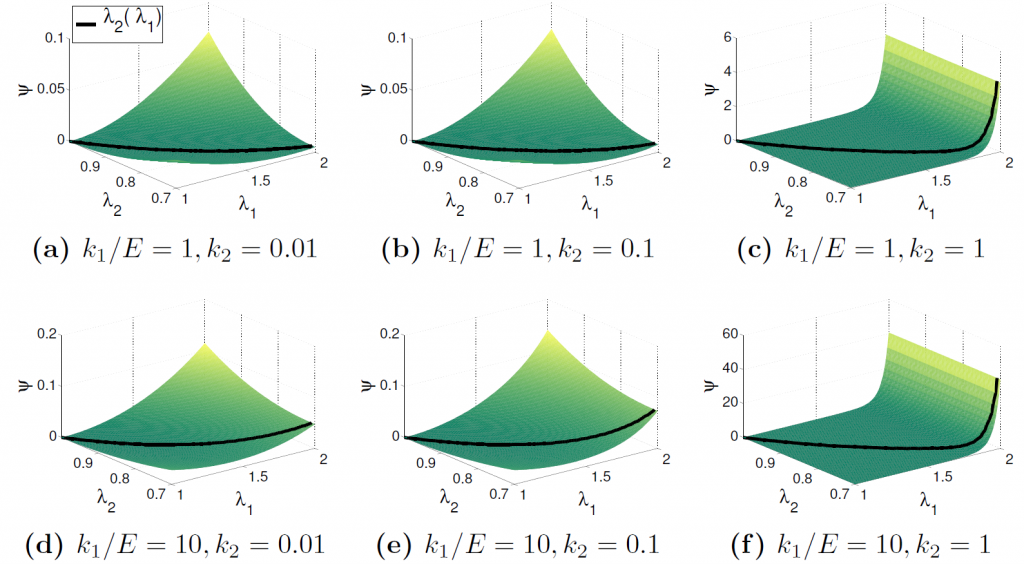Figure 2. Influence of the material parameters on the total strain energy \mathrm{\psi(}\mathrm{\lambda}_\mathrm{1}\mathrm{,}\mathrm{\lambda}_\mathrm{2} using \mathrm{I}_\mathrm{4}; the curves represent the change of \mathrm{\lambda}_\mathrm{2} according to \mathrm{\lambda}_\mathrm{1}.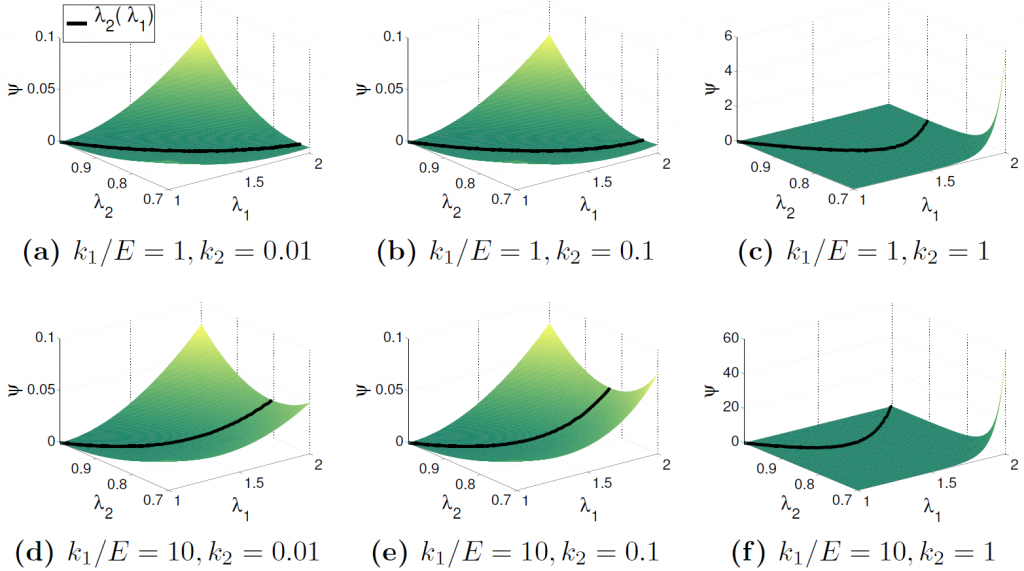Figure 3. Influence of the material parameters on the total strain energy\mathrm{\psi(}\mathrm{\lambda}_\mathrm{1}\mathrm{,}\mathrm{\lambda}_\mathrm{2} using \bar{\mathrm{I}_\mathrm{4}}; the curves represent the change of \mathrm{\lambda}_\mathrm{2} according to \mathrm{\lambda}_\mathrm{1}.

In the above relationship, $\mathrm{\psi}_{\mathrm{aniso}}\left(\mathrm{\lambda}_\mathrm{1}^\mathrm{*}\mathrm{,} \mathrm{\lambda}_\mathrm{2}\right)$ takes the form of Equation (5) or (8), depending on whether the volumetric-deviatoric split is performed on the anisotropic term or not. By means of solving Equation (15) via Newton-Raphson iterations, the value of $\mathrm{\lambda}_\mathrm{2}$ corresponding to each fixed $\mathrm{\lambda}_\mathrm{1}^\mathrm{*}$ can be acquired.

Recall that $\mathrm{E}$ represents the Young’s modulus of the matrix, and $\mathrm{k}_\mathrm{1}$ is the stress-like parameter for the fiber. The ratio between $\mathrm{k}_\mathrm{1}$ and $\mathrm{E}$ captures the level of the reinforcing effect introduced by the fiber. The dimensionless parameter $\mathrm{k}_\mathrm{2}$ of the fiber influences the nonlinearity of the stress-strain relationship. Figures 2 and 3 show the influence of the material parameters $\mathrm{k}_\mathrm{1}\mathrm{/E}$ and $\mathrm{k}_\mathrm{2}$ on the surfaces of the total strain energy $\mathrm{\psi}\left(\mathrm{\lambda}_\mathrm{1}\mathrm{,} \mathrm{\lambda}_\mathrm{2}\right)$ using $\mathrm{I}_\mathrm{4}$ and $\bar{\mathrm{I}_\mathrm{4}}$ in the anisotropic term. The curves in these two figures represent the change of $\mathrm{\lambda}_\mathrm{2}$ according to $\mathrm{\lambda}_\mathrm{1}$.

Figures 4 and 5 show the projections of the $\mathrm{\lambda}_\mathrm{2}\mathrm{(}\mathrm{\lambda}_\mathrm{1}\mathrm{)}$ curves on the $\mathrm{\lambda}_\mathrm{2}\mathrm{-}\mathrm{\lambda}_\mathrm{1}$ plane. When $\mathrm{I}_\mathrm{4}$ is used in the anisotropic strain energy, $\mathrm{\lambda}_\mathrm{2}\mathrm{(}\mathrm{\lambda}_\mathrm{1}\mathrm{)}$ is independent of $\mathrm{k}_\mathrm{1}\mathrm{/E}$ and $\mathrm{k}_\mathrm{2}$, as shown in Figure 4a. Also, the material near incompressibility is satisfied, as shown in Figure 4b. On the other hand, when $\bar{\mathrm{I}_\mathrm{4}}$ is used in the anisotropic strain energy, $\mathrm{\lambda}_\mathrm{2}\mathrm{(}\mathrm{\lambda}_\mathrm{1}\mathrm{)}$ varies significantly according to $\mathrm{k}_\mathrm{1}\mathrm{/E}$ and $\mathrm{k}_\mathrm{2}$. As shown in Figure 5a, when the nonlinearity is strong (large $\mathrm{k}_\mathrm{2}$), the stretch ratio $\mathrm{\lambda}_\mathrm{2}$ firstly decreases then increases as $\mathrm{\lambda}_\mathrm{1}$ increases, indicating the expansion of the sample cross-section after initial shrinking. This phenomenon happens because the nearly incompressible condition is violated, especially when the reinforcing effect of the fiber or the nonlinearity is strong (large $\mathrm{k}_\mathrm{1}\mathrm{/E}$ and $\mathrm{k}_\mathrm{2}$), leading to the unrealistic increase of the material volume, see Figure 5b.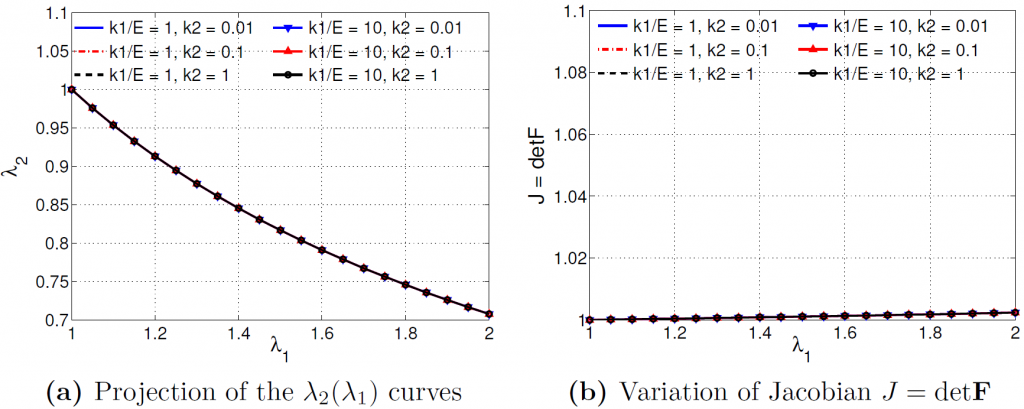Figure 4. Influence of the material parameters on the \mathrm{\lambda}_\mathrm{2}\mathrm{(}\mathrm{\lambda}_\mathrm{1}\mathrm{)} curve and Jacobian \mathrm{J=detF} when \mathrm{I}_\mathrm{4} is used in the anisotropic strain energy.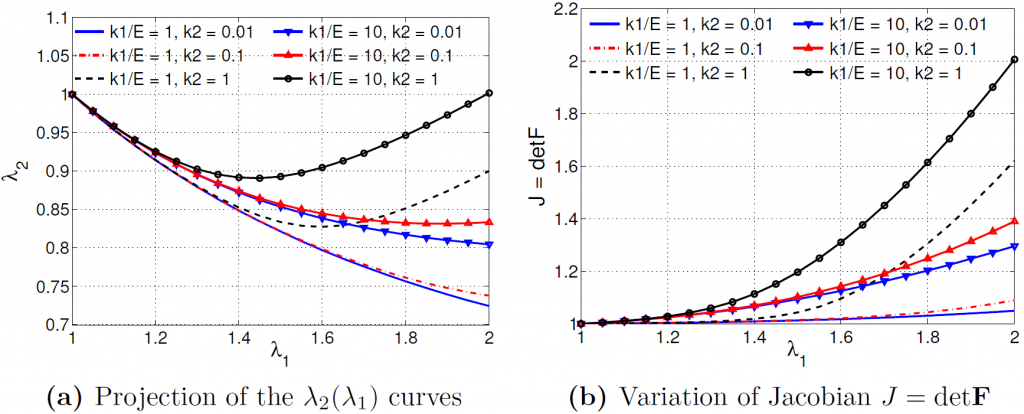Figure 5. Influence of the material parameters on the \mathrm{\lambda}_\mathrm{2}\mathrm{(}\mathrm{\lambda}_\mathrm{1}\mathrm{)} curve and Jacobian \mathrm{J=detF} when \bar{\mathrm{I}_\mathrm{4}} is used in the anisotropic strain energy.

### 3.2. Comparison with the reference solution

The effects of using $\mathrm{I}_\mathrm{4}$ or $\bar{\mathrm{I}_\mathrm{4}}$ in the anisotropic strain energy are further investigated by comparing the results from the $\mathrm{I}_\mathrm{4}$ and $\bar{\mathrm{I}_\mathrm{4}}$ based HGO models with the reference solution, which is obtained from a FE simulation using the embedded fiber approach. The material sample under the same boundary conditions as shown in Figure 1 is used. The ground matrix is described by the modified neo-Hookean law with Young’s modulus $\mathrm{E}$ = 1.0 × 10-3 MPa and Poisson’s ratio ν = 0.499. The mechanical behavior of the embedded fiber is described by an exponential strain energy function

$\mathrm{\psi}_\mathrm{f}\left(\mathrm{\lambda}\right)\mathrm{=}\frac{\mathrm{1}}{\mathrm{2b}}\mathrm{kL}_\mathrm{0}^\mathrm{2}\left(\mathrm{e}^{\mathrm{b}{\mathrm{(}\mathrm{\lambda-1)}}^\mathrm{2}}-1\right),$

(16)

where the axial stiffness $\mathrm{k=0.013}$ MPa and the dimensionless parameter $\mathrm{b=0.7}$. The numerical result obtained from the embedded fiber approach is used as the reference solution, which is compared with the results from the HGO model using $\mathrm{I}_\mathrm{4}$ and $\bar{\mathrm{I}_\mathrm{4}}$ in the anisotropic term respectively.
Both the HGO model and the embedded fiber approach use the same material parameters $\mathrm{E}$ and ν for the isotropic matrix term. The material parameters $\left(\mathrm{k}_\mathrm{1}\mathrm{,} \mathrm{k}_\mathrm{2}\right)$ for the anisotropic term in the HGO model are calibrated according to the force-stretch relationship $\mathrm{f}^\mathrm{ref}\mathrm {(\lambda}_\mathrm{1}\mathrm{)}$ of the reference solution. For the $\mathrm{I}_\mathrm{4}$ based HGO model, the optimal values of $\left(\mathrm{k}_\mathrm{1}\mathrm{,} \mathrm{k}_\mathrm{2}\right)$ are obtained as follows,

$\left(\mathrm{k}_\mathrm{1}\mathrm{,} \mathrm{k}_\mathrm{2}\right)\mathrm{=}\underset{\mathrm{k}_\mathrm{1}\mathrm{,} \mathrm{k}_\mathrm{2}\mathrm{\in(0,+\infty)}}{\mathrm{arg}{\mathrm{\ min}}}||\mathrm{f}^{\mathrm{I}_\mathrm{4}}\left(\mathrm{k}_\mathrm{1}\mathrm{,} \mathrm{k}_\mathrm{2}\mathrm{,}\mathrm{\lambda}_\mathrm{1}\right)\mathrm{-f}^\mathrm{ref}\left(\mathrm{\lambda}_\mathrm{1}\right)||_\mathrm{2}.$

(17)

The optimal values of $\left(\mathrm{k}_\mathrm{1}\mathrm{,} \mathrm{k}_\mathrm{2}\right)$ for the$\bar{\mathrm{I}_\mathrm{4}}$ based HGO model can be acquired via a similar approach. Figures 6 and 7 show the calibrations of $\left(\mathrm{k}_\mathrm{1}\mathrm{,} \mathrm{k}_\mathrm{2}\right)$ for the $\mathrm{I}_\mathrm{4}$ and $\bar{\mathrm{I}_\mathrm{4}}$ based HGO models. Table 1 shows the material parameters used in different modeling approaches.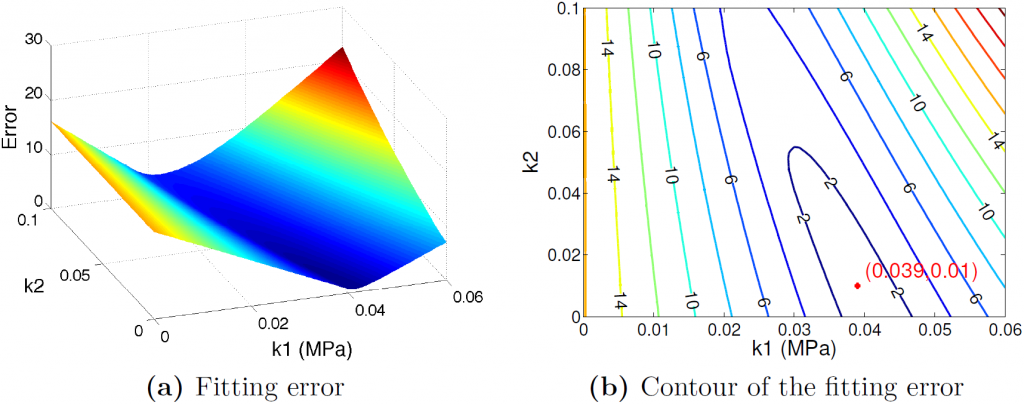Figure 6. Calibration of \mathrm{k}_\mathrm{1} and \mathrm{k}_\mathrm{2} for the anisotropic term using \mathrm{I}_\mathrm{4}; minimum error is achieved at (0.039,0.01).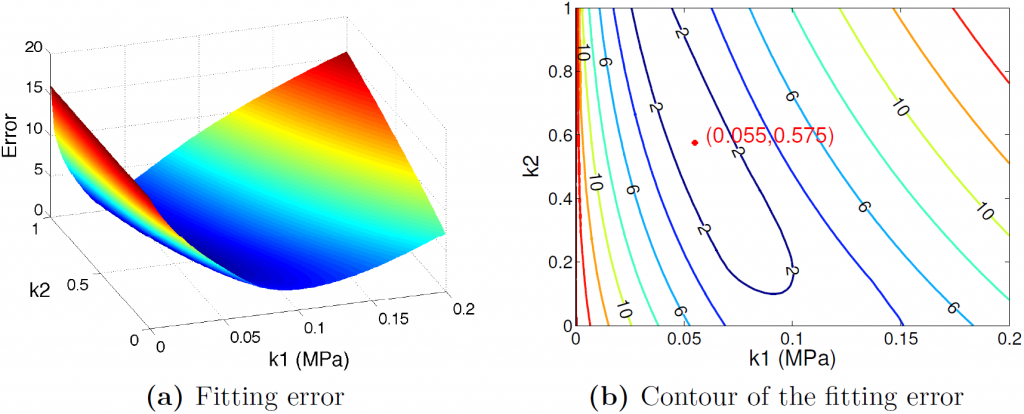Figure 7. Calibration of \mathrm{k}_\mathrm{1} and \mathrm{k}_\mathrm{2} for the anisotropic term using \bar{\mathrm{I}_\mathrm{4}}; minimum error is achieved at (0.055,0.575).

The force-stretch curves obtained from the $\mathrm{I}_\mathrm{4}$ and $\bar{\mathrm{I}_\mathrm{4}}$ based HGO models both match the reference solution, as shown in Figure 8. Figure 9a shows that when $\mathrm{I}_\mathrm{4}$ is used in the anisotropic term, the transverse stretch ratio $\mathrm{\lambda}_\mathrm{2}$ monotonically decreases as $\mathrm{\lambda}_\mathrm{1}$ increases, and the $\mathrm{\lambda}_\mathrm{1}\mathrm{(}\mathrm{\lambda}_\mathrm{2}\mathrm{)}$ curve matches the counterpart of the reference solution. However, when $\bar{\mathrm{I}_\mathrm{4}}$ is used in the anisotropic term, the transverse stretch ratio $\mathrm{\lambda}_\mathrm{2}$ first decreases then increases, indicating a contraction followed by later expansion of the cross-section. This non-physical growth of material volume when $\bar{\mathrm{I}_\mathrm{4}}$ is used in the anisotropic strain energy violates the material nearly incompressible condition, as shown in Figure 9b.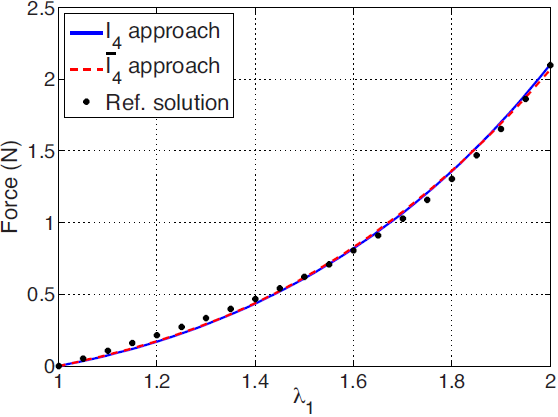Figure 8. Comparison of the force-stretch curves using \mathrm{I}_\mathrm{4} and \bar{\mathrm{I}_\mathrm{4}} in the anisotropic term with the reference solution.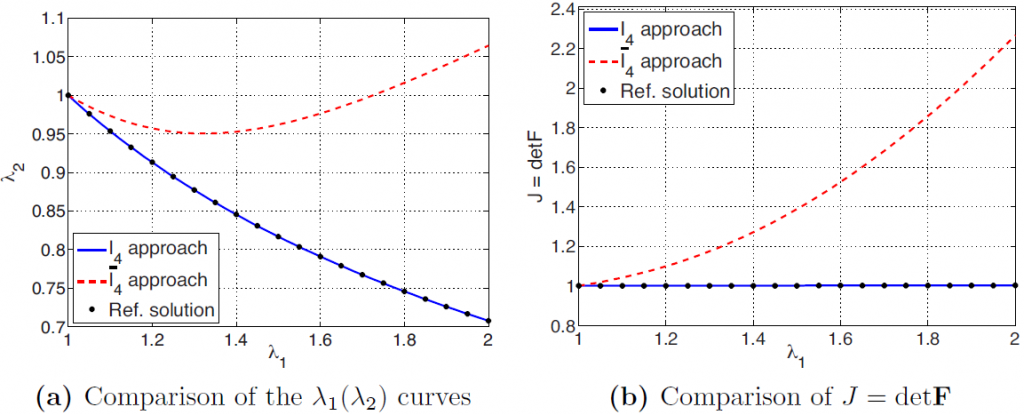Figure 9. Comparison of the \mathrm{\lambda}_\mathrm{1}\mathrm{(}\mathrm{\lambda}_\mathrm{2}\mathrm{)} curves and Jacobian \mathrm{J=detF} using \mathrm{I}_\mathrm{4} and \bar{\mathrm{I}_\mathrm{4}} in the anisotropic term with the reference solution.

## 4. Conclusions

In this research, we combine theoretical analysis and numerical results to straightforwardly demonstrate that the volumetric-deviatoric split should not be performed on the anisotropic (fiber) strain energy for fiber-reinforced materials. Specifically, we construct an intuitive example and analyze the influence of $\mathrm{I}_\mathrm{4}$ and $\bar{\mathrm{I}_\mathrm{4}}$ on the HGO model behavior. We show that when $\mathrm{I}_\mathrm{4}$ is adopted in the anisotropic strain energy, the transverse stretch ratio of the sample monotonically decreases. This is because the material near incompressibility is well preserved during the deformation. Since the fiber does not contribute to the transverse stresses, the transverse deformation only depends on the isotropic (matrix) strain energy. This phenomenon fits with the underlying assumption that fibers act as one-dimensional components and reinforce the material only along the fiber orientations. On the other hand, when$\bar{\mathrm{I}_\mathrm{4}}$ is used in the anisotropic strain energy, the transverse stretch ratio $\mathrm{\lambda}_\mathrm{2}$ first decreases then increases, indicating a contraction followed by later expansion of the cross-section. The dependence of the sample transverse deformation on the anisotropic strain energy can be explained by the expression of the Cauchy stress. When$\bar{\mathrm{I}_\mathrm{4}}$ is used, the fiber counter-intuitively acts as a multi-dimensional component and contributes to the transverse stresses. We further compare the numerical results from the $\mathrm{I}_\mathrm{4}$ and $\bar{\mathrm{I}_\mathrm{4}}$based HGO models with the reference solution, which is obtained via the embedded fiber approach. Although the force-stretch relationships both match the reference solution, only the HGO model using $\mathrm{I}_\mathrm{4}$ in the anisotropic term also matches the transverse deformation of the reference solution. The HGO model using$\bar{\mathrm{I}_\mathrm{4}}$ exhibits non-physical volume growth and violates the material nearly incompressibility condition. Based on the analytical and numerical results presented in this research, we consolidate the conclusion that in the numerical simulation of fiber-reinforced nearly incompressible materials, the volumetric-deviatoric split should not be performed on the anisotropic strain energy and the full pseudo-invariant $\mathrm{I}_\mathrm{4}$ of the structural tensor should be used.

## References

1.            Holzapfel GA, Gasser TC, Ogden RW. A New Constitutive Framework for Arterial Wall Mechanics and a Comparative Study of Material Models. Journal of elasticity and the physical science of solids. 2000;61(1):1-48. https://doi.org/10.1023/A:1010835316564.

2.            Bischoff JE, Arruda EM, Grosh K. Finite element modeling of human skin using an isotropic, nonlinear elastic constitutive model. J Biomech. 2000;33(6):645-52. https://doi.org/10.1016/s0021-9290(00)00018-x.

3.            Balzani D, Neff P, Schröder J, Holzapfel GA. A polyconvex framework for soft biological tissues. Adjustment to experimental data. International journal of solids and structures. 2006;43(20):6052-70. https://doi.org/10.1016/j.ijsolstr.2005.07.048.

4.            Gasser TC, Ogden RW, Holzapfel GA. Hyperelastic modelling of arterial layers with distributed collagen fibre orientations. J R Soc Interface. 2006;3(6):15-35. https://doi.org/10.1098/rsif.2005.0073.

5.            D’Amore A, Stella JA, Wagner WR, Sacks MS. Characterization of the complete fiber network topology of planar fibrous tissues and scaffolds. Biomaterials. 2010;31(20):5345-54. https://doi.org/10.1016/j.biomaterials.2010.03.052.

6.            D’Amore A, Amoroso N, Gottardi R, Hobson C, Carruthers C, Watkins S, et al. From single fiber to macro-level mechanics: A structural finite-element model for elastomeric fibrous biomaterials. J Mech Behav Biomed Mater. 2014;39:146-61. https://doi.org/10.1016/j.jmbbm.2014.07.016.

7.            Holzapfel G, Mechanics N-lS. A continuum approach for engineering. John Wiley and Sons, Chichester, UK; 2000.

8.            Bonet J, Wood R. J. Bonet, R. D. Wood, Nonlinear Continuum Mechanics for Finite Element Analysis, Cambridge University Press, Cambridge, UK2008.

9.            Bonet J, Burton AJ. A simple orthotropic, transversely isotropic hyperelastic constitutive equation for large strain computations. Computer Methods in Applied Mechanics and Engineering. 1998;162(1):151-64. https://doi.org/10.1016/S0045-7825(97)00339-3.

10.          Itskov M, Aksel N. A Class of Orthotropic and Transversely Isotropic Hyperelastic Constitutive Models Based on a Polyconvex Strain Energy Function. International Journal of Solids and Structures. 2004;41:3833–48. https://doi.org/10.1016/j.ijsolstr.2004.02.027.

11.          Ehret AE, Itskov M. A polyconvex hyperelastic model for fiber-reinforced materials in application to soft tissues. Journal of Materials Science. 2007;42(21):8853-63. https://doi.org/10.1007/s10853-007-1812-6.

12.          Schröder J, Neff P, Balzani D. A variational approach for materially stable anisotropic hyperelasticity. International journal of solids and structures. 2005;42(15):4352-71. https://doi.org/10.1016/j.ijsolstr.2004.11.021.

13.          Pandolfi A, Vasta M. Fiber distributed hyperelastic modeling of biological tissues. Mechanics of Materials. 2012;44:151-62. https://doi.org/10.1016/j.mechmat.2011.06.004.

14.          Sansour C. On the physical assumptions underlying the volumetric-isochoric split and the case of anisotropy. European Journal of Mechanics-A/Solids. 2008;27(1):28-39. https://doi.org/10.1016/j.euromechsol.2007.04.001. 15.          Helfenstein J, Jabareen M, Mazza E, Govindjee S. On non-physical response in models for fiber-reinforced hyperelastic materials. International Journal of Solids and Structures. 2010;47(16):2056-61. https://doi.org/10.1016/j.ijsolstr.2010.04.005.